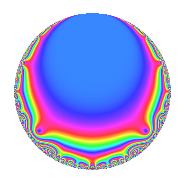# Properties

 Label 62.2.c.bLevel 62 Weight 2 Character orbit 62.c Analytic conductor 0.495 Analytic rank 0 Dimension 2 CM No Inner twists 2

# Related objects

## Newspace parameters

 Level: $$N$$ = $$62 = 2 \cdot 31$$ Weight: $$k$$ = $$2$$ Character orbit: $$[\chi]$$ = 62.c (of order $$3$$ and degree $$2$$)

## Newform invariants

 Self dual: No Analytic conductor: $$0.495072492532$$ Analytic rank: $$0$$ Dimension: $$2$$ Coefficient field: $$\Q(\sqrt{-3})$$ Coefficient ring: $$\Z[a_1, \ldots, a_{5}]$$ Coefficient ring index: $$1$$ Sato-Tate group: $\mathrm{SU}(2)[C_{3}]$

## $q$-expansion

Coefficients of the $$q$$-expansion are expressed in terms of a primitive root of unity $$\zeta_{6}$$. We also show the integral $$q$$-expansion of the trace form.

 $$f(q)$$ $$=$$ $$q + q^{2} + ( -3 + 3 \zeta_{6} ) q^{3} + q^{4} -\zeta_{6} q^{5} + ( -3 + 3 \zeta_{6} ) q^{6} + ( 3 - 3 \zeta_{6} ) q^{7} + q^{8} -6 \zeta_{6} q^{9} +O(q^{10})$$ $$q + q^{2} + ( -3 + 3 \zeta_{6} ) q^{3} + q^{4} -\zeta_{6} q^{5} + ( -3 + 3 \zeta_{6} ) q^{6} + ( 3 - 3 \zeta_{6} ) q^{7} + q^{8} -6 \zeta_{6} q^{9} -\zeta_{6} q^{10} + 3 \zeta_{6} q^{11} + ( -3 + 3 \zeta_{6} ) q^{12} -5 \zeta_{6} q^{13} + ( 3 - 3 \zeta_{6} ) q^{14} + 3 q^{15} + q^{16} + ( -3 + 3 \zeta_{6} ) q^{17} -6 \zeta_{6} q^{18} + ( -7 + 7 \zeta_{6} ) q^{19} -\zeta_{6} q^{20} + 9 \zeta_{6} q^{21} + 3 \zeta_{6} q^{22} -4 q^{23} + ( -3 + 3 \zeta_{6} ) q^{24} + ( 4 - 4 \zeta_{6} ) q^{25} -5 \zeta_{6} q^{26} + 9 q^{27} + ( 3 - 3 \zeta_{6} ) q^{28} + 2 q^{29} + 3 q^{30} + ( 5 - 6 \zeta_{6} ) q^{31} + q^{32} -9 q^{33} + ( -3 + 3 \zeta_{6} ) q^{34} -3 q^{35} -6 \zeta_{6} q^{36} + ( -1 + \zeta_{6} ) q^{37} + ( -7 + 7 \zeta_{6} ) q^{38} + 15 q^{39} -\zeta_{6} q^{40} + 9 \zeta_{6} q^{41} + 9 \zeta_{6} q^{42} + ( 1 - \zeta_{6} ) q^{43} + 3 \zeta_{6} q^{44} + ( -6 + 6 \zeta_{6} ) q^{45} -4 q^{46} -8 q^{47} + ( -3 + 3 \zeta_{6} ) q^{48} -2 \zeta_{6} q^{49} + ( 4 - 4 \zeta_{6} ) q^{50} -9 \zeta_{6} q^{51} -5 \zeta_{6} q^{52} + 3 \zeta_{6} q^{53} + 9 q^{54} + ( 3 - 3 \zeta_{6} ) q^{55} + ( 3 - 3 \zeta_{6} ) q^{56} -21 \zeta_{6} q^{57} + 2 q^{58} + ( -3 + 3 \zeta_{6} ) q^{59} + 3 q^{60} + 6 q^{61} + ( 5 - 6 \zeta_{6} ) q^{62} -18 q^{63} + q^{64} + ( -5 + 5 \zeta_{6} ) q^{65} -9 q^{66} + 3 \zeta_{6} q^{67} + ( -3 + 3 \zeta_{6} ) q^{68} + ( 12 - 12 \zeta_{6} ) q^{69} -3 q^{70} + \zeta_{6} q^{71} -6 \zeta_{6} q^{72} -7 \zeta_{6} q^{73} + ( -1 + \zeta_{6} ) q^{74} + 12 \zeta_{6} q^{75} + ( -7 + 7 \zeta_{6} ) q^{76} + 9 q^{77} + 15 q^{78} + ( -1 + \zeta_{6} ) q^{79} -\zeta_{6} q^{80} + ( -9 + 9 \zeta_{6} ) q^{81} + 9 \zeta_{6} q^{82} -5 \zeta_{6} q^{83} + 9 \zeta_{6} q^{84} + 3 q^{85} + ( 1 - \zeta_{6} ) q^{86} + ( -6 + 6 \zeta_{6} ) q^{87} + 3 \zeta_{6} q^{88} + 6 q^{89} + ( -6 + 6 \zeta_{6} ) q^{90} -15 q^{91} -4 q^{92} + ( 3 + 15 \zeta_{6} ) q^{93} -8 q^{94} + 7 q^{95} + ( -3 + 3 \zeta_{6} ) q^{96} + 14 q^{97} -2 \zeta_{6} q^{98} + ( 18 - 18 \zeta_{6} ) q^{99} +O(q^{100})$$ $$\operatorname{Tr}(f)(q)$$ $$=$$ $$2q + 2q^{2} - 3q^{3} + 2q^{4} - q^{5} - 3q^{6} + 3q^{7} + 2q^{8} - 6q^{9} + O(q^{10})$$ $$2q + 2q^{2} - 3q^{3} + 2q^{4} - q^{5} - 3q^{6} + 3q^{7} + 2q^{8} - 6q^{9} - q^{10} + 3q^{11} - 3q^{12} - 5q^{13} + 3q^{14} + 6q^{15} + 2q^{16} - 3q^{17} - 6q^{18} - 7q^{19} - q^{20} + 9q^{21} + 3q^{22} - 8q^{23} - 3q^{24} + 4q^{25} - 5q^{26} + 18q^{27} + 3q^{28} + 4q^{29} + 6q^{30} + 4q^{31} + 2q^{32} - 18q^{33} - 3q^{34} - 6q^{35} - 6q^{36} - q^{37} - 7q^{38} + 30q^{39} - q^{40} + 9q^{41} + 9q^{42} + q^{43} + 3q^{44} - 6q^{45} - 8q^{46} - 16q^{47} - 3q^{48} - 2q^{49} + 4q^{50} - 9q^{51} - 5q^{52} + 3q^{53} + 18q^{54} + 3q^{55} + 3q^{56} - 21q^{57} + 4q^{58} - 3q^{59} + 6q^{60} + 12q^{61} + 4q^{62} - 36q^{63} + 2q^{64} - 5q^{65} - 18q^{66} + 3q^{67} - 3q^{68} + 12q^{69} - 6q^{70} + q^{71} - 6q^{72} - 7q^{73} - q^{74} + 12q^{75} - 7q^{76} + 18q^{77} + 30q^{78} - q^{79} - q^{80} - 9q^{81} + 9q^{82} - 5q^{83} + 9q^{84} + 6q^{85} + q^{86} - 6q^{87} + 3q^{88} + 12q^{89} - 6q^{90} - 30q^{91} - 8q^{92} + 21q^{93} - 16q^{94} + 14q^{95} - 3q^{96} + 28q^{97} - 2q^{98} + 18q^{99} + O(q^{100})$$

## Character Values

We give the values of $$\chi$$ on generators for $$\left(\mathbb{Z}/62\mathbb{Z}\right)^\times$$.

 $$n$$ $$3$$ $$\chi(n)$$ $$-\zeta_{6}$$

## Embeddings

For each embedding $$\iota_m$$ of the coefficient field, the values $$\iota_m(a_n)$$ are shown below.

For more information on an embedded modular form you can click on its label.

Label $$\iota_m(\nu)$$ $$a_{2}$$ $$a_{3}$$ $$a_{4}$$ $$a_{5}$$ $$a_{6}$$ $$a_{7}$$ $$a_{8}$$ $$a_{9}$$ $$a_{10}$$
5.1
 0.5 + 0.866025i 0.5 − 0.866025i
1.00000 −1.50000 + 2.59808i 1.00000 −0.500000 0.866025i −1.50000 + 2.59808i 1.50000 2.59808i 1.00000 −3.00000 5.19615i −0.500000 0.866025i
25.1 1.00000 −1.50000 2.59808i 1.00000 −0.500000 + 0.866025i −1.50000 2.59808i 1.50000 + 2.59808i 1.00000 −3.00000 + 5.19615i −0.500000 + 0.866025i
 $$n$$: e.g. 2-40 or 990-1000 Significant digits: Format: Complex embeddings Normalized embeddings Satake parameters Satake angles

## Inner twists

Char. orbit Parity Mult. Self Twist Proved
1.a Even 1 trivial yes
31.c Even 1 yes

## Hecke kernels

This newform can be constructed as the kernel of the linear operator $$T_{3}^{2} + 3 T_{3} + 9$$ acting on $$S_{2}^{\mathrm{new}}(62, [\chi])$$.